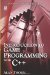# Chapter 3: The Pictures of Mathematics

## Overview

The first chapter studied the basics of mathematics and the second continued to explore its application in algebra. This chapter harnesses knowledge from previous chapters and examines specifically how mathematics relates to space, shapes, movement, and distance. In order to understand this we must examine a number of mathematical branches in this chapter. None of these can be explored in their entirety, but all of them must be touched upon so far as is relevant to programming and especially to game making. These branches include to some degree geometry, trigonometry, graph theory, and linear algebra. Specifically, this chapter examines the following subjects:

• Coordinate systems

• Primitives

• Vertices, rays, and lines

• Angles, degrees, and radians

• Squares, circles, and triangles

• Vectors

• Sine, cosine, tangent, and other trigonometric ratiosIntroduction to Game Programming with C++ (Wordware Game Developers Library)
ISBN: 1598220322
EAN: 2147483647
Year: 2007
Pages: 225
Authors: Alan Thorn

Similar book on Amazon

flylib.com © 2008-2017.
If you may any questions please contact us: flylib@qtcs.net